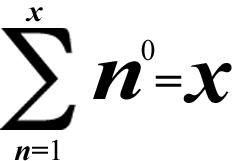# Super Summation 4## Example:1^0 + 2^0 + 3^0 + 4^0 + 5^0 + 6^0 + 7^0 + 8^0 + 9^0 = 1 + 1 + 1 + 1 + 1 + 1 + 1 + 1 + 1 = 1 × 9 = 9

(1 is the number of multiplicative identity, so n × 1 = n; notice that there are 9 1's!)

The caret (^) is the symbol of exponentiation. Raising a non-zero number to the power of zero(0) always gives you 1.

You can only pick counting numbers to be x in this summation formula because 1 is the number below the sigma, to the right of the small n & equal sign. All positive integers are counting numbers. (By the way, zero(0) isn't a counting number because it's neither positive nor negative; it's neutral)